# Design of Gear Box

29. Sep 2016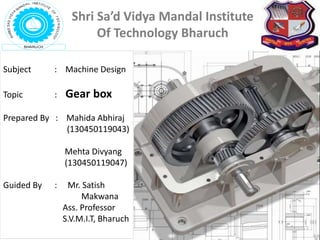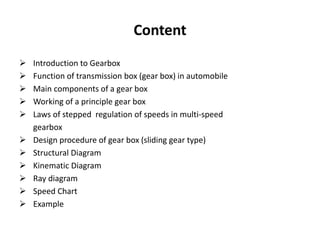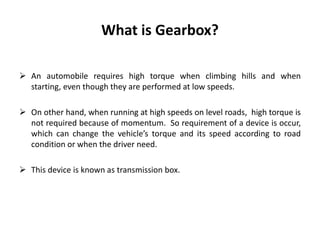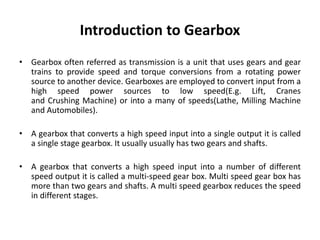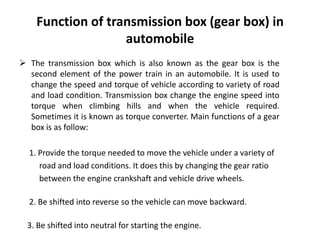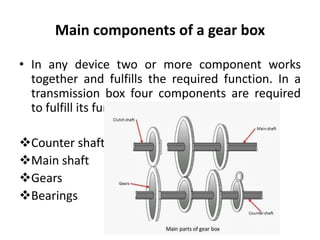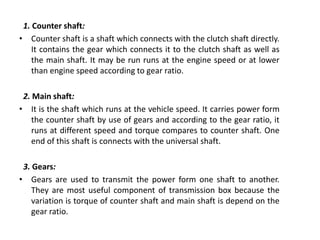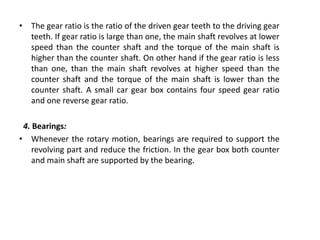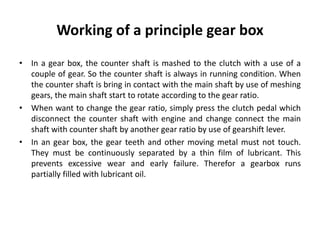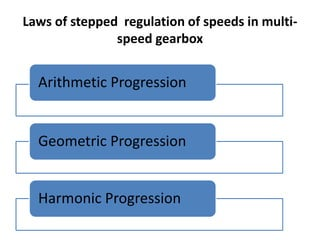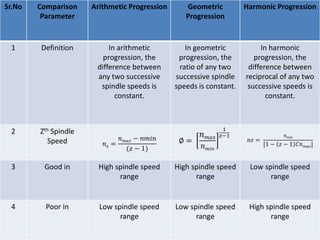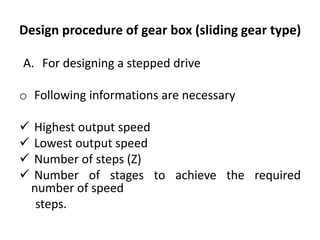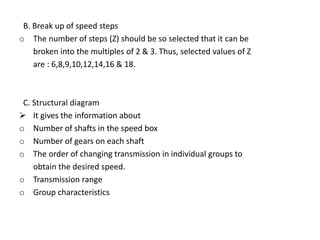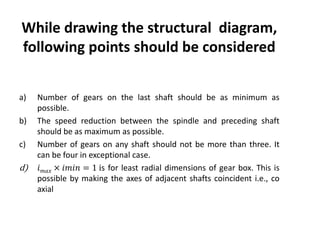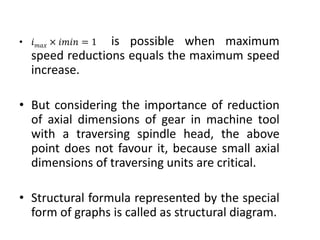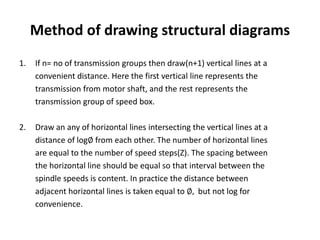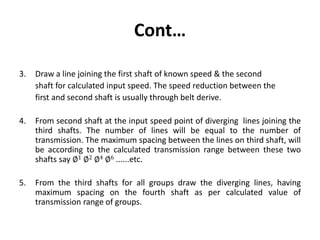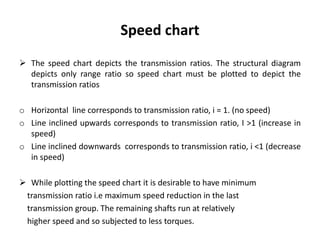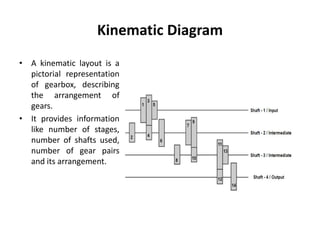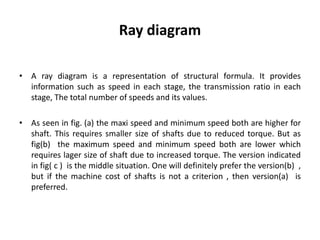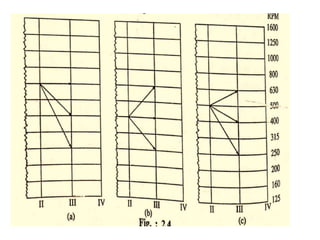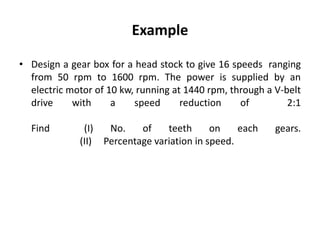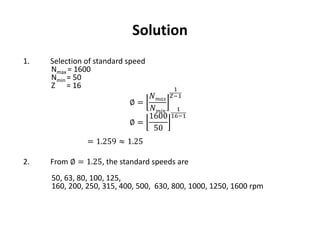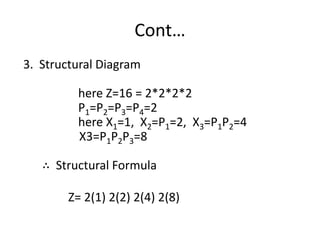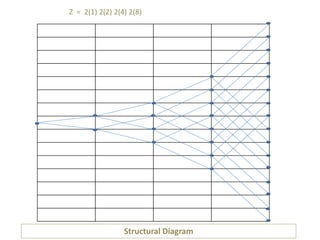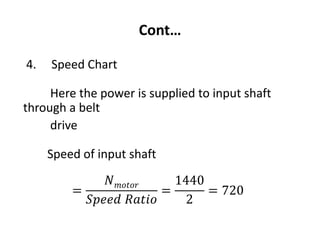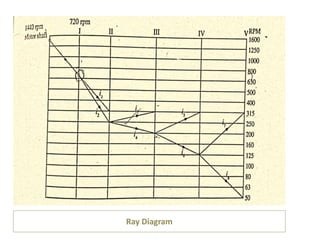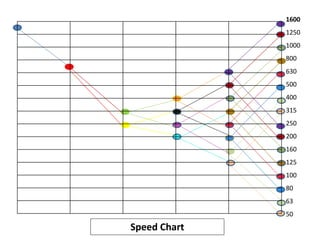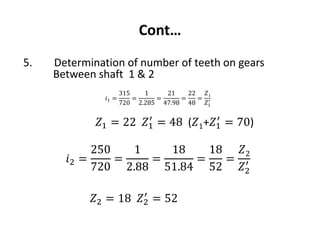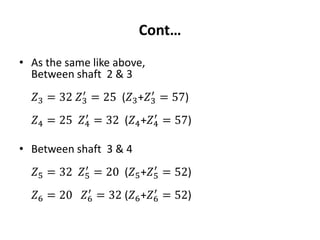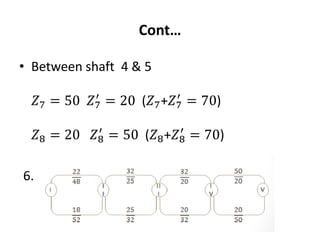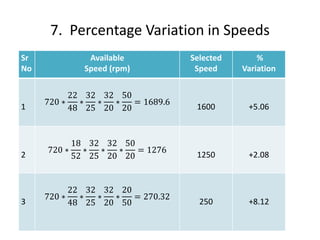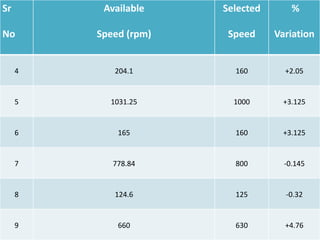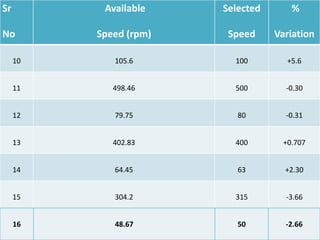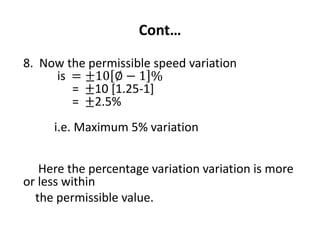1 von 36

### Design of Gear Box

• 1. Subject : Machine Design Topic : Gear box Prepared By : Mahida Abhiraj (130450119043) Mehta Divyang (130450119047) Guided By : Mr. Satish Makwana Ass. Professor S.V.M.I.T, Bharuch Shri Sa’d Vidya Mandal Institute Of Technology Bharuch
• 2. Content  Introduction to Gearbox  Function of transmission box (gear box) in automobile  Main components of a gear box  Working of a principle gear box  Laws of stepped regulation of speeds in multi-speed gearbox  Design procedure of gear box (sliding gear type)  Structural Diagram  Kinematic Diagram  Ray diagram  Speed Chart  Example
• 3. What is Gearbox?  An automobile requires high torque when climbing hills and when starting, even though they are performed at low speeds.  On other hand, when running at high speeds on level roads, high torque is not required because of momentum. So requirement of a device is occur, which can change the vehicle’s torque and its speed according to road condition or when the driver need.  This device is known as transmission box.
• 4. Introduction to Gearbox • Gearbox often referred as transmission is a unit that uses gears and gear trains to provide speed and torque conversions from a rotating power source to another device. Gearboxes are employed to convert input from a high speed power sources to low speed(E.g. Lift, Cranes and Crushing Machine) or into a many of speeds(Lathe, Milling Machine and Automobiles). • A gearbox that converts a high speed input into a single output it is called a single stage gearbox. It usually usually has two gears and shafts. • A gearbox that converts a high speed input into a number of different speed output it is called a multi-speed gear box. Multi speed gear box has more than two gears and shafts. A multi speed gearbox reduces the speed in different stages.
• 5. Function of transmission box (gear box) in automobile  The transmission box which is also known as the gear box is the second element of the power train in an automobile. It is used to change the speed and torque of vehicle according to variety of road and load condition. Transmission box change the engine speed into torque when climbing hills and when the vehicle required. Sometimes it is known as torque converter. Main functions of a gear box is as follow: 1. Provide the torque needed to move the vehicle under a variety of road and load conditions. It does this by changing the gear ratio between the engine crankshaft and vehicle drive wheels. 2. Be shifted into reverse so the vehicle can move backward. 3. Be shifted into neutral for starting the engine.
• 6. Main components of a gear box • In any device two or more component works together and fulfills the required function. In a transmission box four components are required to fulfill its function. Counter shaft Main shaft Gears Bearings
• 7. 1. Counter shaft: • Counter shaft is a shaft which connects with the clutch shaft directly. It contains the gear which connects it to the clutch shaft as well as the main shaft. It may be run runs at the engine speed or at lower than engine speed according to gear ratio. 2. Main shaft: • It is the shaft which runs at the vehicle speed. It carries power form the counter shaft by use of gears and according to the gear ratio, it runs at different speed and torque compares to counter shaft. One end of this shaft is connects with the universal shaft. 3. Gears: • Gears are used to transmit the power form one shaft to another. They are most useful component of transmission box because the variation is torque of counter shaft and main shaft is depend on the gear ratio.
• 8. • The gear ratio is the ratio of the driven gear teeth to the driving gear teeth. If gear ratio is large than one, the main shaft revolves at lower speed than the counter shaft and the torque of the main shaft is higher than the counter shaft. On other hand if the gear ratio is less than one, than the main shaft revolves at higher speed than the counter shaft and the torque of the main shaft is lower than the counter shaft. A small car gear box contains four speed gear ratio and one reverse gear ratio. 4. Bearings: • Whenever the rotary motion, bearings are required to support the revolving part and reduce the friction. In the gear box both counter and main shaft are supported by the bearing.
• 9. Working of a principle gear box • In a gear box, the counter shaft is mashed to the clutch with a use of a couple of gear. So the counter shaft is always in running condition. When the counter shaft is bring in contact with the main shaft by use of meshing gears, the main shaft start to rotate according to the gear ratio. • When want to change the gear ratio, simply press the clutch pedal which disconnect the counter shaft with engine and change connect the main shaft with counter shaft by another gear ratio by use of gearshift lever. • In an gear box, the gear teeth and other moving metal must not touch. They must be continuously separated by a thin film of lubricant. This prevents excessive wear and early failure. Therefor a gearbox runs partially filled with lubricant oil.
• 10. Laws of stepped regulation of speeds in multi- speed gearbox Arithmetic Progression Geometric Progression Harmonic Progression
• 11. Sr.No Comparison Parameter Arithmetic Progression Geometric Progression Harmonic Progression 1 Definition In arithmetic progression, the difference between any two successive spindle speeds is constant. In geometric progression, the ratio of any two successive spindle speeds is constant. In harmonic progression, the difference between reciprocal of any two successive speeds is constant. 2 Zth Spindle Speed 𝑛 𝑧 = 𝑛 𝑚𝑎𝑥 − 𝑛𝑚𝑖𝑛 (𝑧 − 1) ∅ = 𝑛 𝑚𝑎𝑥 𝑛 𝑚𝑖𝑛 1 𝑧−1 𝑛𝑧 = 𝑛 𝑚𝑖𝑛 [1 − 𝑧 − 1 𝐶𝑛 𝑚𝑎𝑥] 3 Good in High spindle speed range High spindle speed range Low spindle speed range 4 Poor in Low spindle speed range Low spindle speed range High spindle speed range
• 12. Design procedure of gear box (sliding gear type) A. For designing a stepped drive o Following informations are necessary  Highest output speed  Lowest output speed  Number of steps (Z)  Number of stages to achieve the required number of speed steps.
• 13. B. Break up of speed steps o The number of steps (Z) should be so selected that it can be broken into the multiples of 2 & 3. Thus, selected values of Z are : 6,8,9,10,12,14,16 & 18. C. Structural diagram  It gives the information about o Number of shafts in the speed box o Number of gears on each shaft o The order of changing transmission in individual groups to obtain the desired speed. o Transmission range o Group characteristics
• 14. While drawing the structural diagram, following points should be considered a) Number of gears on the last shaft should be as minimum as possible. b) The speed reduction between the spindle and preceding shaft should be as maximum as possible. c) Number of gears on any shaft should not be more than three. It can be four in exceptional case. d) 𝑖 𝑚𝑎𝑥 × 𝑖𝑚𝑖𝑛 = 1 is for least radial dimensions of gear box. This is possible by making the axes of adjacent shafts coincident i.e., co axial
• 15. • 𝑖 𝑚𝑎𝑥 × 𝑖𝑚𝑖𝑛 = 1 is possible when maximum speed reductions equals the maximum speed increase. • But considering the importance of reduction of axial dimensions of gear in machine tool with a traversing spindle head, the above point does not favour it, because small axial dimensions of traversing units are critical. • Structural formula represented by the special form of graphs is called as structural diagram.
• 16. Method of drawing structural diagrams 1. If n= no of transmission groups then draw(n+1) vertical lines at a convenient distance. Here the first vertical line represents the transmission from motor shaft, and the rest represents the transmission group of speed box. 2. Draw an any of horizontal lines intersecting the vertical lines at a distance of log∅ from each other. The number of horizontal lines are equal to the number of speed steps(Z). The spacing between the horizontal line should be equal so that interval between the spindle speeds is content. In practice the distance between adjacent horizontal lines is taken equal to ∅, but not log for convenience.
• 17. Cont… 3. Draw a line joining the first shaft of known speed & the second shaft for calculated input speed. The speed reduction between the first and second shaft is usually through belt derive. 4. From second shaft at the input speed point of diverging lines joining the third shafts. The number of lines will be equal to the number of transmission. The maximum spacing between the lines on third shaft, will be according to the calculated transmission range between these two shafts say ∅1 ∅2 ∅4 ∅6 ......etc. 5. From the third shafts for all groups draw the diverging lines, having maximum spacing on the fourth shaft as per calculated value of transmission range of groups.
• 18. Speed chart  The speed chart depicts the transmission ratios. The structural diagram depicts only range ratio so speed chart must be plotted to depict the transmission ratios o Horizontal line corresponds to transmission ratio, i = 1. (no speed) o Line inclined upwards corresponds to transmission ratio, I >1 (increase in speed) o Line inclined downwards corresponds to transmission ratio, i <1 (decrease in speed)  While plotting the speed chart it is desirable to have minimum transmission ratio i.e maximum speed reduction in the last transmission group. The remaining shafts run at relatively higher speed and so subjected to less torques.
• 19. Kinematic Diagram • A kinematic layout is a pictorial representation of gearbox, describing the arrangement of gears. • It provides information like number of stages, number of shafts used, number of gear pairs and its arrangement.
• 20. Ray diagram • A ray diagram is a representation of structural formula. It provides information such as speed in each stage, the transmission ratio in each stage, The total number of speeds and its values. • As seen in fig. (a) the maxi speed and minimum speed both are higher for shaft. This requires smaller size of shafts due to reduced torque. But as fig(b) the maximum speed and minimum speed both are lower which requires lager size of shaft due to increased torque. The version indicated in fig( c ) is the middle situation. One will definitely prefer the version(b) , but if the machine cost of shafts is not a criterion , then version(a) is preferred.
• 22. Example • Design a gear box for a head stock to give 16 speeds ranging from 50 rpm to 1600 rpm. The power is supplied by an electric motor of 10 kw, running at 1440 rpm, through a V-belt drive with a speed reduction of 2:1 Find (I) No. of teeth on each gears. (II) Percentage variation in speed.
• 23. Solution 1. Selection of standard speed Nmax = 1600 Nmin = 50 Z = 16 ∅ = 𝑁 𝑚𝑎𝑥 𝑁 𝑚𝑖𝑛 1 𝑍−1 ∅ = 1600 50 1 16−1 = 1.259 ≈ 1.25 2. From ∅ = 1.25, the standard speeds are 50, 63, 80, 100, 125, 160, 200, 250, 315, 400, 500, 630, 800, 1000, 1250, 1600 rpm
• 24. Cont… 3. Structural Diagram here Z=16 = 2*2*2*2 P1=P2=P3=P4=2 here X1=1, X2=P1=2, X3=P1P2=4 X3=P1P2P3=8 ∴ Structural Formula Z= 2(1) 2(2) 2(4) 2(8)
• 25. Structural Diagram Z = 2(1) 2(2) 2(4) 2(8)
• 26. Cont… 4. Speed Chart Here the power is supplied to input shaft through a belt drive Speed of input shaft = 𝑁 𝑚𝑜𝑡𝑜𝑟 𝑆𝑝𝑒𝑒𝑑 𝑅𝑎𝑡𝑖𝑜 = 1440 2 = 720
• 27. Ray Diagram
• 28. 1600 1250 1000 800 630 500 400 315 250 200 160 125 100 80 63 50 Speed Chart
• 29. Cont… 5. Determination of number of teeth on gears Between shaft 1 & 2 𝑖1 = 315 720 = 1 2.285 = 21 47.98 = 22 48 = 𝑍1 𝑍1 ′ 𝑍1 = 22 𝑍1 ′ = 48 (𝑍1+𝑍1 ′ = 70) 𝑖2 = 250 720 = 1 2.88 = 18 51.84 = 18 52 = 𝑍2 𝑍2 ′ 𝑍2 = 18 𝑍2 ′ = 52
• 30. Cont… • As the same like above, Between shaft 2 & 3 𝑍3 = 32 𝑍3 ′ = 25 (𝑍3+𝑍3 ′ = 57) 𝑍4 = 25 𝑍4 ′ = 32 (𝑍4+𝑍4 ′ = 57) • Between shaft 3 & 4 𝑍5 = 32 𝑍5 ′ = 20 (𝑍5+𝑍5 ′ = 52) 𝑍6 = 20 𝑍6 ′ = 32 (𝑍6+𝑍6 ′ = 52)
• 31. Cont… • Between shaft 4 & 5 𝑍7 = 50 𝑍7 ′ = 20 (𝑍7+𝑍7 ′ = 70) 𝑍8 = 20 𝑍8 ′ = 50 (𝑍8+𝑍8 ′ = 70) 6. Schematic Representation of drive
• 32. 7. Percentage Variation in Speeds Sr No Available Speed (rpm) Selected Speed % Variation 1 720 ∗ 22 48 ∗ 32 25 ∗ 32 20 ∗ 50 20 = 1689.6 1600 +5.06 2 720 ∗ 18 52 ∗ 32 25 ∗ 32 20 ∗ 50 20 = 1276 1250 +2.08 3 720 ∗ 22 48 ∗ 32 25 ∗ 32 20 ∗ 20 50 = 270.32 250 +8.12
• 33. Sr No Available Speed (rpm) Selected Speed % Variation 4 204.1 160 +2.05 5 1031.25 1000 +3.125 6 165 160 +3.125 7 778.84 800 -0.145 8 124.6 125 -0.32 9 660 630 +4.76
• 34. Sr No Available Speed (rpm) Selected Speed % Variation 10 105.6 100 +5.6 11 498.46 500 -0.30 12 79.75 80 -0.31 13 402.83 400 +0.707 14 64.45 63 +2.30 15 304.2 315 -3.66 16 48.67 50 -2.66
• 35. Cont… 8. Now the permissible speed variation is = ±10 ∅ − 1 % = ±10 [1.25-1] = ±2.5% i.e. Maximum 5% variation Here the percentage variation variation is more or less within the permissible value.
• 36. Thank You